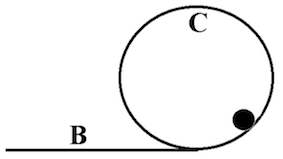# Problem: A cylinder with mass m, radius a, and moment of inertia with respect to the center of mass ICM = 1/2 ma2, rolls without slipping around a loop with radius R as shown in the figure. a) What is the minimum speed of the center of mass at point C (vC) for the cylinder to move around the loop without falling off? b) What is the total kinetic energy of the cylinder at point C in the case of minimum speed? c) What is the minimum speed of the center of mass necessary at point B (vB) for the cylinder to move around the loop without falling off at the top (point C)? Write your results in terms of a, R, m, and g. Check the units/dimensions for each answer.

###### FREE Expert Solution
83% (454 ratings)
###### Problem Details

A cylinder with mass m, radius a, and moment of inertia with respect to the center of mass ICM = 1/2 ma2, rolls without slipping around a loop with radius R as shown in the figure.

a) What is the minimum speed of the center of mass at point C (vC) for the cylinder to move around the loop without falling off?

b) What is the total kinetic energy of the cylinder at point C in the case of minimum speed?

c) What is the minimum speed of the center of mass necessary at point B (vB) for the cylinder to move around the loop without falling off at the top (point C)?

Write your results in terms of a, R, m, and g. Check the units/dimensions for each answer.Frequently Asked Questions

What scientific concept do you need to know in order to solve this problem?

Our tutors have indicated that to solve this problem you will need to apply the Energy of Rolling Motion concept. You can view video lessons to learn Energy of Rolling Motion. Or if you need more Energy of Rolling Motion practice, you can also practice Energy of Rolling Motion practice problems.

How long does this problem take to solve?

Our expert Physics tutor, Jeffery took 10 minutes and 5 seconds to solve this problem. You can follow their steps in the video explanation above.

What professor is this problem relevant for?

Based on our data, we think this problem is relevant for Professor Lockett-Ruiz's class at CSUF.